Question

THEOREM 205. Define the functions fr : [0, 1] + R by Sn(:1) = x" /n...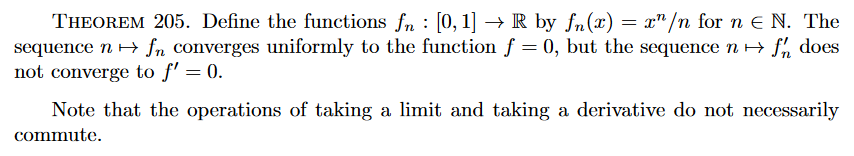THEOREM 205. Define the functions fr : [0, 1] + R by Sn(:1) = x" /n for n E N. The sequence n H Sn converges uniformly to the function f = 0, but the sequence n o fh does not converge to f' = 0. Note that the operations of taking a limit and taking a derivative do not necessarily commute.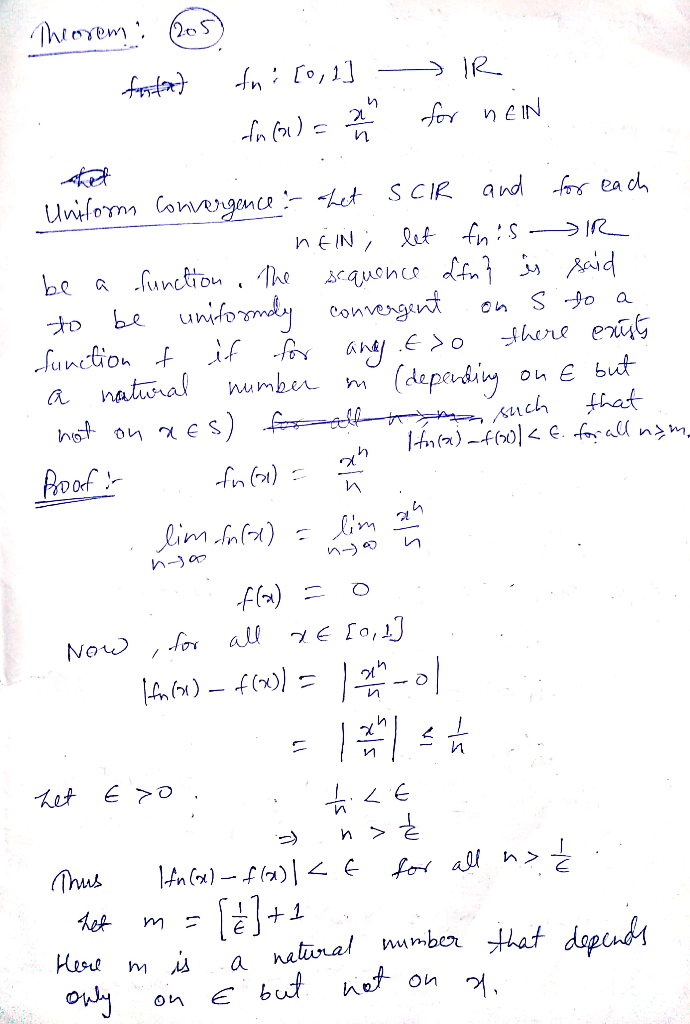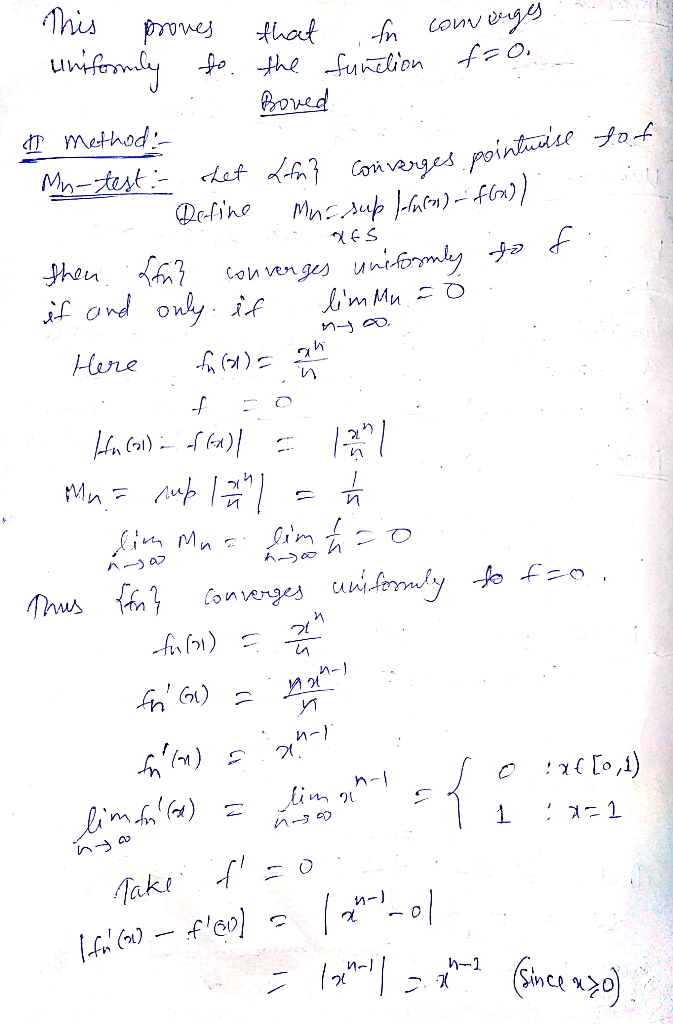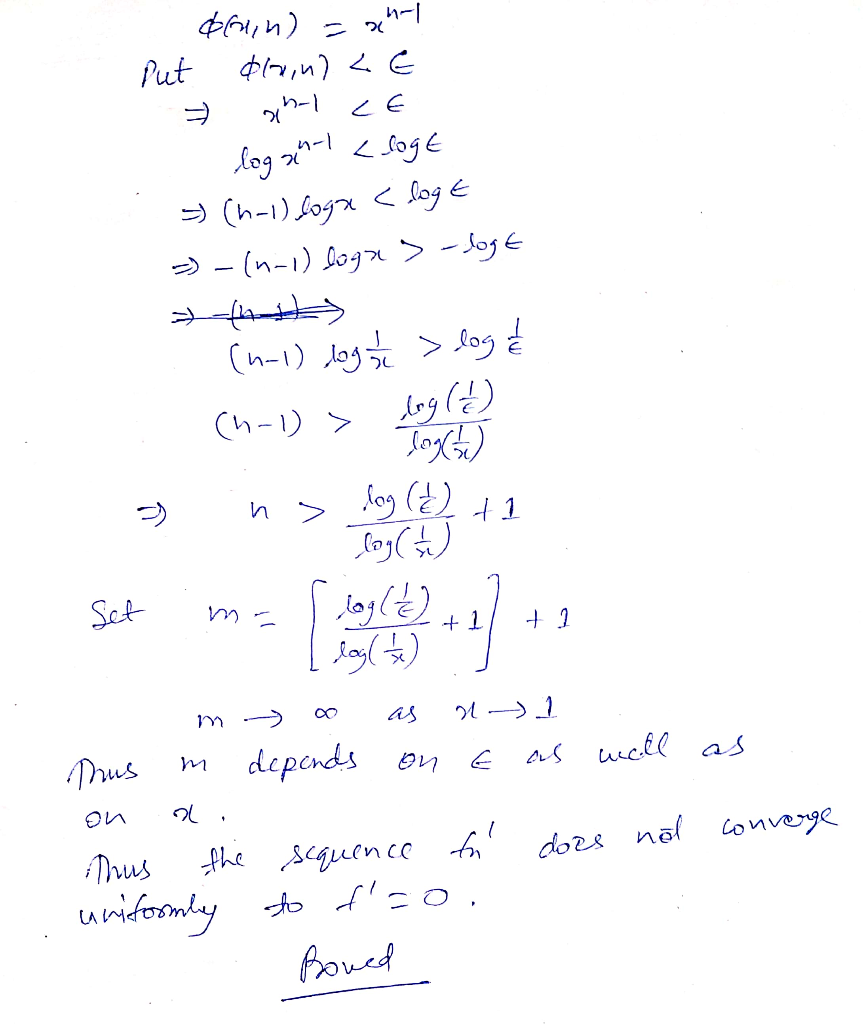Earn Coins

Coins can be redeemed for fabulous gifts.

Similar Homework Help Questions
• = 5a. (10 pts) Let fr : [0, 1] → R, fn(x) ce-nzº, for m =...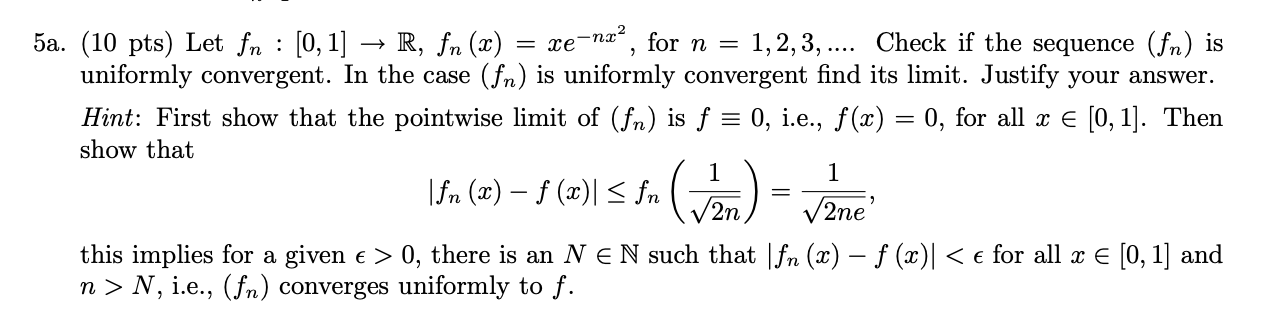= 5a. (10 pts) Let fr : [0, 1] → R, fn(x) ce-nzº, for m = = 1, 2, 3, .... Check if the sequence (fn) is uniformly convergent. In the case (fr) is uniformly convergent find its limit. Justify your answer. Hint: First show that the pointwise limit of (fr) is f = 0, i.e., f (x) = 0, for all x € [0, 1]. Then show that 1 \Sn (r) – 5 (w) SS, (cm) - Vžne 1...

• 4. Suppose (fr)nen is a sequence of functions on [0, 1] such that each fn is...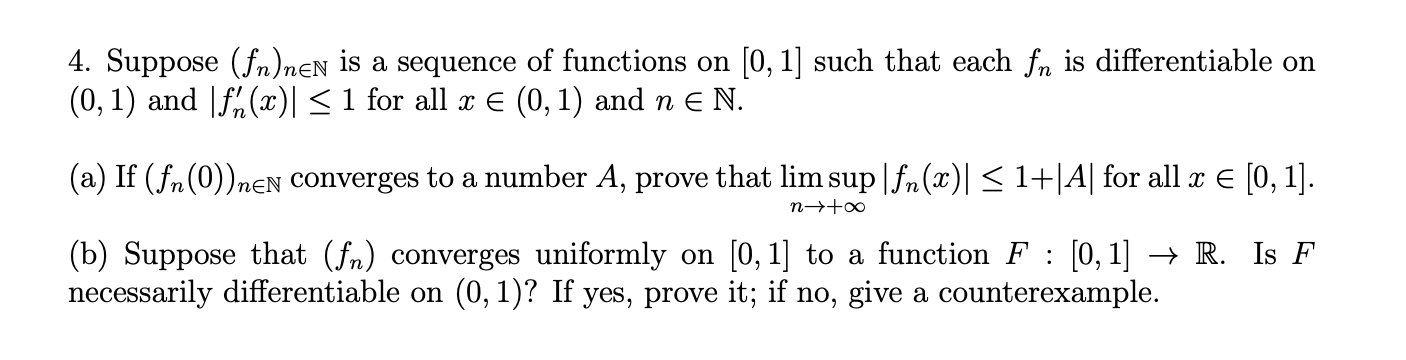4. Suppose (fr)nen is a sequence of functions on [0, 1] such that each fn is differentiable on (0,1) and f(x) < 1 for all x € (0,1) and n e N. (a) If (fn (0))nen converges to a number A, prove that lim sup|fn(x) = 1+|A| for all x € [0, 1]. n-too : (b) Suppose that (fr) converges uniformly on [0, 1] to a function F : [0, 1] + R. Is F necessarily differentiable on (0,1)? If...

• For each n E N, define a function fn A - R. Suppose that each function fn is uniformly continuous. Moreover, suppose there is a function f : A R such that for all є 0, there exists a N, and for all x...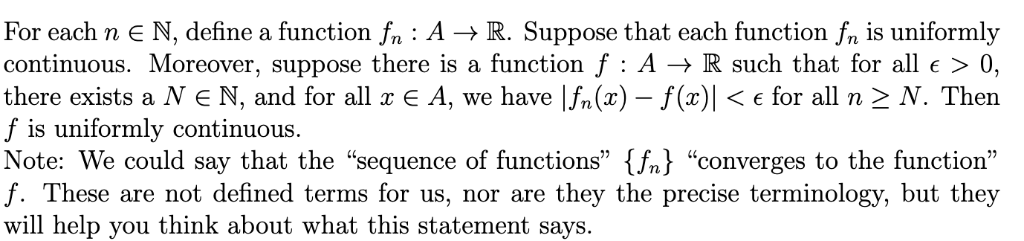For each n E N, define a function fn A - R. Suppose that each function fn is uniformly continuous. Moreover, suppose there is a function f : A R such that for all є 0, there exists a N, and for all x E A, we have lÍs(x)-f(x)|く for all n > N. Then f is uniformly continuous. Note: We could say that the "sequence of functions" f "converges to the function" f. These are not defined terms for...

• 5. Let fn(x) = x"/n on [0, 1]. Show that (fr)nen converges uniformly to a differentiable...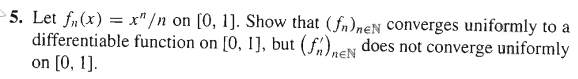5. Let fn(x) = x"/n on [0, 1]. Show that (fr)nen converges uniformly to a differentiable function on [0, 1], but (f%) does not converge uniformly neN on [0, 1].

• I'm not good at front of advanced math because I'm in the middle of the class....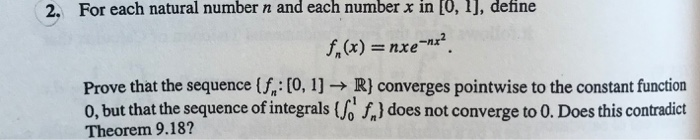I'm not good at front of advanced math because I'm in the middle of the class. If you show me a rigorously detailed proof, I'd like to ask a additional question probably... 2. For each natural numbern and each number x in [0, 1), define f,(x) nxe Prove that the sequence (f: [0, 1] R} converges pointwise to the constant function 0, but that the sequence of integrals (of,) does not converge to 0. Does this contradict Theorem 9.18? THEOREM...

• (h) Define f : [0, 2] + R by 122 if 0 <<<1 f(x) = {...(h) Define f : [0, 2] + R by 122 if 0 <<<1 f(x) = { ifl<152 Using the limit definition of the derivative and the sequence definition of the limit prove that f'(1) does not exist.

• 1. (a) Let {fn}neN : [0,00) + R be a sequence of function define by: sin(nx)...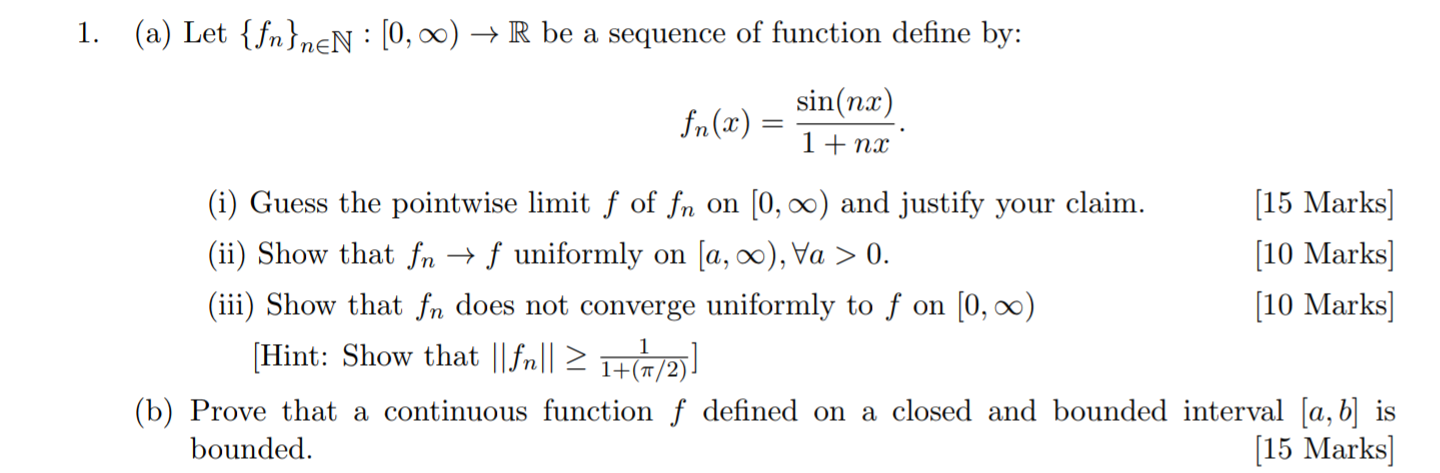1. (a) Let {fn}neN : [0,00) + R be a sequence of function define by: sin(nx) fn(x) 1+ nx (i) Guess the pointwise limit f of fn on (0,00) and justify your claim. [15 Marks] (ii) Show that fn + f uniformly on ſa, 00), Va > 0. [10 Marks) (iii) Show that fn does not converge uniformly to f on (0,00) [10 Marks] (Hint: Show that ||fr|| 21+(1/2) (b) Prove that a continuous function f defined on a closed...

• 4. Define the functions fn : 1-1, 1] → R given by TL Prove that fn...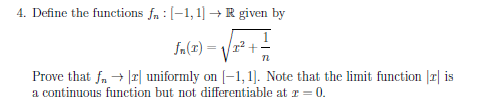4. Define the functions fn : 1-1, 1] → R given by TL Prove that fn → Irl uniformly on I-1, 1]. Note that the limit function Irl is a continuous function but not differentiable at r-0.

• 2. (8 points) Let {fn}n>ı be a sequence of functions that are defined on R by...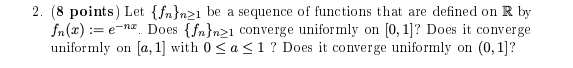2. (8 points) Let {fn}n>ı be a sequence of functions that are defined on R by fn(x):= e-nx. Does {{n}n>1 converge uniformly on [0, 1]? Does it converge uniformly on (a, 1) with 0 <a<1? Does it converge uniformly on (0, 1)?

• (4) Let(an}n=o be a sequence in C. Define R-i-lim suplanlì/n. Recall that R e [0,x] o0 is the rad...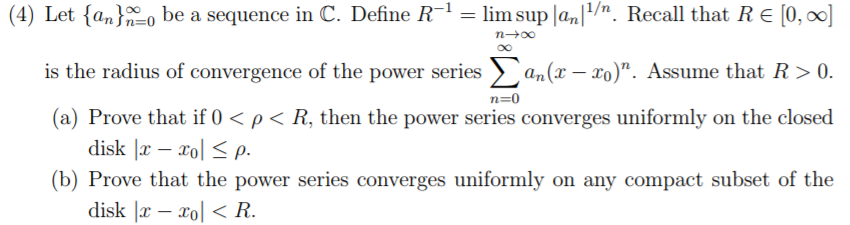(4) Let(an}n=o be a sequence in C. Define R-i-lim suplanlì/n. Recall that R e [0,x] o0 is the radius of convergence of the power series Σ a (z 20)" Assume that R > 0 (a) Prove that if 0 < ρ < R, then the power series converges uniformly on the closed (b) Prove that the power series converges uniformly on any compact subset of the disk Ix - xo< R (4) Let(an}n=o be a sequence in C. Define R-i-lim...